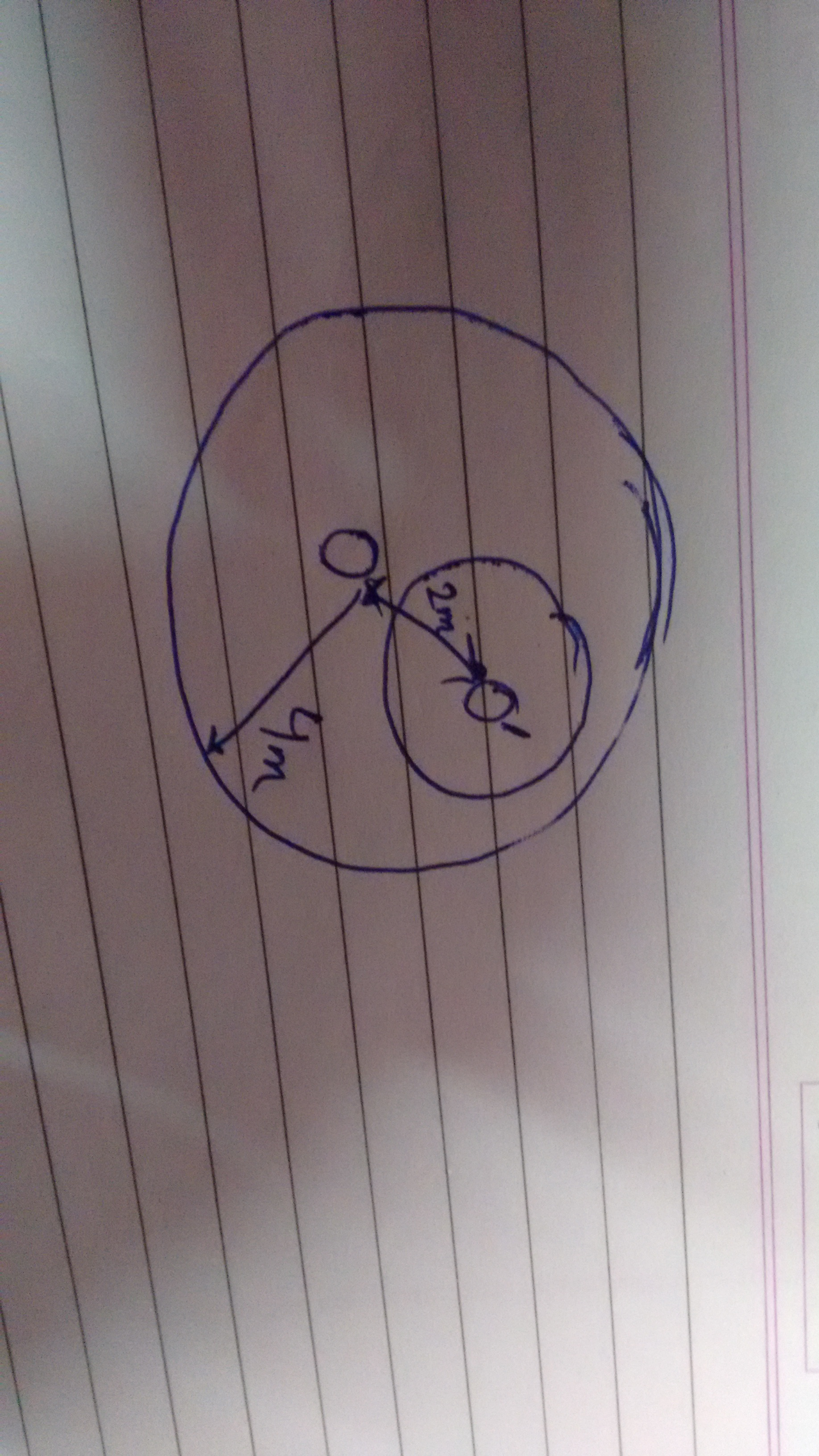# Help me

From a disc of mass 2kg and radius 4m a small disc of radius 1m with center $O^{\prime}$ is extracted .The new moment of inertia about an axis passing through O perpendicular to plane of disc isNote by Kyle Finch
6 years, 4 months ago

This discussion board is a place to discuss our Daily Challenges and the math and science related to those challenges. Explanations are more than just a solution — they should explain the steps and thinking strategies that you used to obtain the solution. Comments should further the discussion of math and science.

When posting on Brilliant:

• Use the emojis to react to an explanation, whether you're congratulating a job well done , or just really confused .
• Ask specific questions about the challenge or the steps in somebody's explanation. Well-posed questions can add a lot to the discussion, but posting "I don't understand!" doesn't help anyone.
• Try to contribute something new to the discussion, whether it is an extension, generalization or other idea related to the challenge.

MarkdownAppears as
*italics* or _italics_ italics
**bold** or __bold__ bold
- bulleted- list
• bulleted
• list
1. numbered2. list
1. numbered
2. list
Note: you must add a full line of space before and after lists for them to show up correctly
paragraph 1paragraph 2

paragraph 1

paragraph 2

[example link](https://brilliant.org)example link
> This is a quote
This is a quote
    # I indented these lines
# 4 spaces, and now they show
# up as a code block.

print "hello world"
# I indented these lines
# 4 spaces, and now they show
# up as a code block.

print "hello world"
MathAppears as
Remember to wrap math in $$ ... $$ or $ ... $ to ensure proper formatting.
2 \times 3 $2 \times 3$
2^{34} $2^{34}$
a_{i-1} $a_{i-1}$
\frac{2}{3} $\frac{2}{3}$
\sqrt{2} $\sqrt{2}$
\sum_{i=1}^3 $\sum_{i=1}^3$
\sin \theta $\sin \theta$
\boxed{123} $\boxed{123}$

Sort by:

- 6 years, 4 months ago

Moment of Inertia of Initial disc about center = Moment of inertia about center of part that is removed+ moment of inertia about center of whatever is left

- 6 years, 4 months ago

I missed the diagram. Plz see above

- 6 years, 4 months ago

I'm still not getting a diagram

- 6 years, 4 months ago

Now u may see

- 6 years, 4 months ago

What I said above still applies. The MOI of the whole disc is sum of the removed part and what's left.

- 6 years, 4 months ago

OK thanks , can u also tell me the solution to this $\frac{dy}{dx}=\frac{x+\sqrt{x^2-4y}}{2}$

- 6 years, 4 months ago